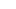# A Data Channel Model for Precognitive Signal Reception Using Multiple Trials by Laurentiu Bucur, Ph.D

February 2, 2014

Intuition

For any N- trials perception process with two equally probable binary outcomes, the following implicit assumption holds:

Each trial j contains two confidence rankings CR1[j] and CR2[j] for each possible outcome. Each sample of the Delta signal CR1[j] – CR2[j] contains a small level of signal observed (in ARV – precognition signal) relative to a superimposing potentially high level of additive noise from multiple sources (environment, judging, etc.). The common intuition and evidence states that nesting multiple trials produces better results in terms of detecting the hidden signal from the noise with a certain confidence level. Below is the framework that shows a mathematical model of such an assumption and gives a simple set of statistical formulas to quantify the confidence level in the detection of the unknown signal after an N-trial perception process, from the raw CR data. The work follows the prevalent assumption in our social groups and in the literature as well as in philosophy that somehow we are able to download information from a noisy data channel, or “tune in” to a noisy universal radio station and pick up subtle signals related to future or past events.

Mathematical method

The following method will provide a confidence level-based formula for precognitive signal detection and the associated direction: positive for Outcome 1, negative for outcome 2.

The core idea is : for a given future target, the resulting Delta signal in each trial is a sample drawn from an underlying stationary process characterized by a normal distribution with a non-zero mean (hidden signal level) and some level of noise sigma2. The sum of k Delta signal samples defines a partial sum signal called the Drift signal which follows a random walk with drift model. The question is when to identify the Drift signal as being above the noise level and to what extent we can say that we have detected a signal component in the raw Drift signal, given a sufficiently large number of trials. The method laid out in this document is based on the calculation of the one tailed Z score relative to SQRT(N)*sigma, with sigma being the quare root of the remote viewing data channel’s variance that is assumed to be a global constant. NOTE: the assumption is that for any target, the variance of the data channel is constant but the signal level is specific to the ARV trial (see Appendix C)

###### Featured PostsNew to APP? How to make predictions

July 16, 2019

1/8

##### APP

© 2016 by Applied Precognition Project